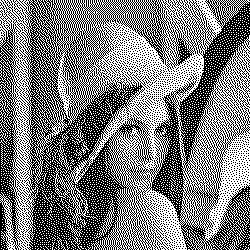Comparing Images

Grafika can compare how similar two images are and can also determine if two images are equal.

Finding Similarity

Finding similarity between two images using the compare() method:

use Grafika\Grafika; // Import package

\$editor = Grafika::createEditor(); // Create editor

\$hammingDistance = \$editor->compare( "image1.jpg", "image-2.jpg" );

The compare method will return a hamming distance. The hamming distance determines how similar two images are. A value of 0 indicates a likely similar picture. A value between 1 and 10 is potentially a variation. A value greater than 10 is likely a different image.

Examples:
Type Image Hamming Distance
Base ImageN/A
Grayscaled0
Dithered0
Smaller,
Watermarked1
Cropped
Note: If you wish to test if an image is cropped try setting the threshold to 26. That means check if the hamming distance is <= 26.23
Totally Different Image35

Checking Equality

Grafika can also do a pixel by pixel comparison to determine if two images are exactly the same using the equal() method: